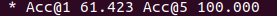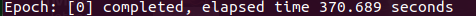# Why add coding at train.py it cannot run?

When adding new coding inside the train.py. The new coding I add is needed to print out the confusion matrix, but after that, the new coding inside the train.py didn’t show anything.
The coding i add inside as shown below:

# %%

import torch
import torchmetrics

def get_Accuracy(num_class):

``````accuracy = torchmetrics.Accuracy(
num_classes=num_class,
average='micro',
multiclass=False
# top_k=1
)

return accuracy
``````

def get_Precision(num_class):

``````precision = torchmetrics.Precision(
num_classes=num_class,
average='micro',
multiclass=False,
)

return precision
``````

def get_Dice():
dice = torchmetrics.Dice(
num_classes=2,
average=‘micro’,

``````)
return dice
``````

# return average_precision

def get_Precision_Recall():
precision_recall = torchmetrics.PrecisionRecallCurve(
num_classes=2,
pos_label=1
)

``````return precision_recall
``````

def get_AUC():
AUC = torchmetrics.AUC(
reorder=True
)
return AUC

def get_F1Score():
F1_Score = torchmetrics.F1Score(
num_classes=2,

``````)
return F1_Score
``````

def get_Confusion_Matrix():
confusion_matrix = torchmetrics.ConfusionMatrix(
num_classes=2,
multilabel=False
)

``````return confusion_matrix
``````

# %%

Hi,

Except confusion matrix, do you get acc and result output log?

Thanks.

NoOnly show this result

Hi,

Which train.py are you using?
Is it the below one? But the source looks very different compared to the content you shared.

Thanks.

I using the jetson inference train.py

There is no update from you for a period, assuming this is not an issue any more.
Hence we are closing this topic. If need further support, please open a new one.
Thanks

Hi,

Could you share which script you are using with us?
Thanks.

This topic was automatically closed 14 days after the last reply. New replies are no longer allowed.